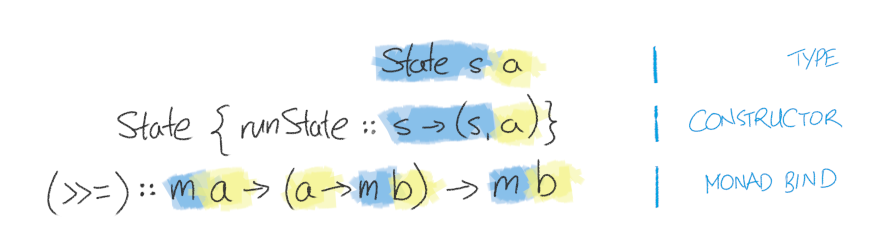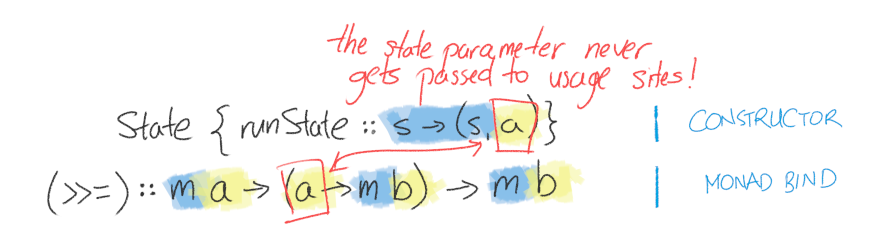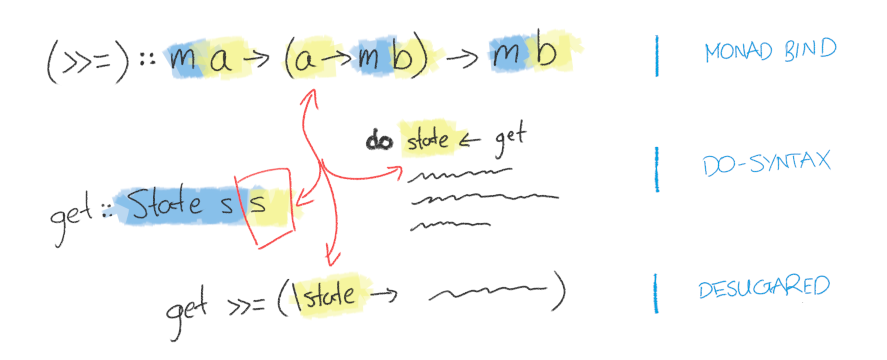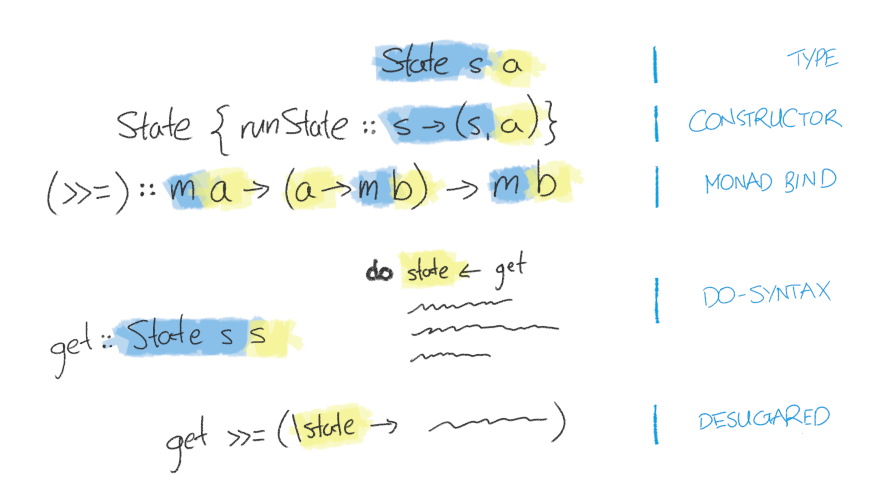# Deriving the State monad from first principles

July 12, 2020

In this series of posts, we’re going to talk about some specific monad instances, the Reader-Writer-State trio, often abbreviated to just `RWS`. We’re actually going to look at them a bit out of order, starting with State.

These posts will be targeted to people who already have some knowledge about monads and what they’re for. Perhaps you’ve never seen these monads before and you’re wondering what’s so special about them. Maybe you vaguely understand that they let you have “mutability” or “global variables” the way you would in other, non-pure languages, but aren’t sure how they work. If you didn’t have these things provided to you, how would you derive them from first principles?

For the proactive who want to learn by doing, this article is designed around you actively working along with the code samples and exercises. So make sure you have an editor and REPL handy while you read this. In fact, if you don’t feel like reading the rest of this article and just want to get some hands-on experience, here’s the skinny version: given the following type signatures, implement the Functor, Applicative, and Monad instances for `State`, as well as the listed function signatures.

``````newtype State s a = State { runState :: s -> (s, a) }

get    :: State s s
put    :: s -> State s ()
modify :: (s -> s) -> State s ()``````

For anyone who wants a little more explanation, let’s dive into it, shall we?

Let’s say that you really, really need some kind of mutability or state. I know, I know; purity good mutation bad. But suppose you’re working on a problem that would be much easier with some kind of mutation, like needing a cache of previously-computed values. Or keeping some kind of count that all levels of your code need to check.

How would we go about doing that in Haskell? Not with some kind of special syntax or variable declaration, clearly. It’s a bit obvious, but also a point worth reiterating: in Haskell, functions only take in inputs and return outputs, and nothing else. So we’ll have to look for a way to simulate statefulness, using just pure functions.

What if we just treated our “variable” as a normal parameter? We’d need each function we write to be able to access it, so our functions will get an extra state parameter. And they’ll also need some way to update it, which, since our functions are pure, must mean that it returns our updated state as an extra value as well.

Let’s say we’re specifically trying to keep a count of how many functions we’ve called. Maybe we end up with something like this:

``````-- Reverse a list, and increase a count of function calls
reverseWithCount :: Int -> [a] -> (Int, [a])
reverseWithCount funcCount list =
(funcCount + 1, reverse list)``````

Note how if we remove the “extraneous” state parameters from the signature, we just get a function of type `[a] -> [a]`, which is exactly the signature of a normal reverse function.

We can then use this function in other definitions:

``````appendReversedWithCount :: Int -> [a] -> [a] -> (Int, [a])
appendReversedWithCount funcCount list1 list2 =
let (funcCount', revList1) = reverseWithCount funcCount list1
(funcCount'', revList2) = reverseWithCount funcCount' list2
in (funcCount'' + 1, revList1 ++ revList2)``````

Exercise 1: Stop here and implement a function `append3ReversedWithCount`, which does the same thing as our append above (reverses the input lists, then appends them in their original order), but for three lists. Use `reverseWithCount`, but not `appendReversedWithCount`, in your definition. Remember that you need to “thread” the state value through all the functions called.

Solution

``````append3ReversedWithCount :: Int -> [a] -> [a] -> [a] -> (Int, [a])
append3ReversedWithCount funcCount list1 list2 list3 =
let (funcCount', revList1) = reverseWithCount funcCount list1
(funcCount'', revList2) = reverseWithCount funcCount' list2
(funcCount''', revList3) = reverseWithCount funcCount'' list3
in (funcCount''' + 1, revList1 ++ revList2 ++ revList3)``````

We did it! Our functions lower in the stack are able to make updates to the count, and those updates get seen by our functions higher in the stack. Now we just pass in some initial state when we want to call one of these functions, like a 0 for the current count, and we’ll get the result we want back out. If our functions need to check the current state to branch off of, they can; it’s just a normal parameter like any other. And this solution “scales”; we can both add more data into our state (by making our state parameter a record or a tuple, by adding more parameters), and we can use our definitions here to build higher- and higher-level functions that also use mutation.

Take that, other languages! We can do mutation too, and it doesn’t even require language support. That’s actually a pretty important point, so let’s say it again: we can have mutation using only pure functions and values.

But clearly this is not a very pleasant solution to use. We’ve had to mangle our function definitions with lots of boilerplate purely to thread the state through, boilerplate that wouldn’t be necessary in a language that had first-class support for mutation. And it’s very easy to make mistakes while writing code like this; maybe you accidentally returned the wrong intermediate state value from one of the many functions you called. Maybe you passed the wrong current state to a function call. Maybe the function you’re calling has another parameter with the same type as your state, and you mixed up the order when calling it. So while this works, it’s not something that we’d like to use in actual code.

The real problem is that adding mutability has made it difficult to call functions. We the programmers are now responsible for threading the updated state values, managing the current state and so on, instead of that bookkeeping being done for us automatically.

Perhaps you have an intuition that monads can allow us to have functions that manage some kind of extra “context” for us. In this case, that “context” is the current state. So maybe looking at this from a monadic perspective can help solve our problem? But for that to work, we need some data structure that’s a monad instance. What would that be in our case?

Let’s take a closer look at the signatures of the functions we’ve written so far. Is there a “core type” that all of our functions have in common, some kind of “essence” of statefulness?

``````reverseWithCount         :: Int -> [a] -> (Int, [a])
appendReversedWithCount  :: Int -> [a] -> [a] -> (Int, [a])
append3ReversedWithCount :: Int -> [a] -> [a] -> [a] -> (Int, [a])``````

Do the list parameters here have anything to do with statefulness? No, they’re specific to each function in question. So we can remove those from our hypothetical core type. What about the list return values? No again, those are specific to each function in question, and we can make those abstract. So we’re left with our Int parameter and return values. And since there’s no reason our state has to be just numeric, we can make those abstract as well. In the end, the type we’re left with is:

``aStatefulFunction :: state -> (state, a)``

That is, the “core” of a stateful function is that it takes in a current state and returns an updated state along with its “normal” return value.

We’ve got a type. Let’s put it inside a data definition and give it a shot.

``data State s a = State { runState :: s -> (s, a) }``

Do we know whether this will form a valid monad just from looking at it? Not really; the only thing to do is try to write the typeclass instances and see if it works and obeys the monad laws.1

But before we dive into implementing, why would doing this transformation and rewriting all our functions to use this datatype even help us in the first place?

Recall the functions that a monad gives you: `return` (or `pure`), and `(>>=)`. Look at their abstract types:

``````return :: Monad m => a -> m a
(>>=)  :: Monad m => m a -> (a -> m b) -> m b``````

How do the types of these functions relate to our actual datatype?In particular, notice that if we use `(>>=)`, we don’t have any way of accessing our current state:If we use `(>>=)` to construct our functions, the current state gets completely abstracted away underneath that ‘`m`’ type parameter.

From one perspective, that sounds kind of backwards from what we’re trying to do, i.e. provide some way of updating the state. But from another perspective, that’s actually exactly what we want to get rid of the boilerplate of threading the state value from earlier. The only thing we can access is whatever “normal” return value we get from the function we’ve called. Whatever `(>>=)` that we write should handle doing the state threading, not us.

As an example, let’s assume that we already have correctly-implemented functor/applicative/monad instances for our new datatype. What might our definitions for our functions from before look like, using the monad functions?

``````reverseWithCount :: [a] -> State Int [a]
reverseWithCount list = State (\s ->
(s + 1, reverse list))

appendReversedWithCount :: [a] -> [a] -> State Int [a]
appendReversedWithCount list1 list2 =
reverseWithCount list1 >>= (\revList1 ->
reverseWithCount list2 >>= (\revList2 ->
State (\s -> (s + 1, revList1 ++ revList2))))

append3ReversedWithCount :: [a] -> [a] -> [a] -> State Int [a]
append3ReversedWithCount list1 list2 list3 =
reverseWithCount list1 >>= (\revList1 ->
reverseWithCount list2 >>= (\revList2 ->
reverseWithCount list3 >>= (\revList3 ->
State (\s -> (s + 1, revList1 ++ revList2 ++ revList3)))))``````

It’s not the prettiest code, and we’ll see how this gets improved later. But even at this stage, notice how almost all of the boilerplate around passing the state parameter has disappeared. We’re left almost entirely with just the logic of reversing and appending lists; the only place where we have to explicitly manage the current state is when we want our current function to make some changes. Instead of us having to manage updates from any functions we call, it looks as though `(>>=)` is doing that for us. So whatever implementation we write for monadic bind, that’s where we move the state handling that previously we were doing manually.

Exercise 2: Implement the Functor, Applicative, and Monad instances for our newly-defined State type.

One thing I find very helpful when writing instances for these classes is to explicitly write out what the type of each typeclass function is supposed to be, when applied to our type. For instance, the type of `fmap` would be:

``fmap :: (a -> b) -> State s a -> State s b``

Hint

The instance for Functor is fairly straightforward, but if you’ve never seen them before, the instances for Applicative and Monad can be a bit tricky. Remember that the return value of both `(<*>)` and `(>>=)` should also be State values; don’t be afraid to construct such values directly. Remember that you can deconstruct the passed-in State values as well. You’re currently implementing the abstractions, not using them, so it’s perfectly fine to work with the “internal” representation.

Keep in mind what we mentioned earlier: the point of `(>>=)` (and similarly, `(<*>)`) is to do the exact same threading of the current state that we previously did manually.

Solution

``````instance Functor (State s) where
fmap f (State stateFn) = State (\s ->
let (s', result) = stateFn s
in (s', f result))

instance Applicative (State s) where
pure x = State (\s -> (s, x))
(<*>) (State stateFx) (State stateX) = State (\s ->
let (s', fx) = stateFx s
(s'', x) = stateX s'
in (s'', fx x))

return = pure
(>>=) (State stateX) nextFn = State (\s ->
let (s', x) = stateX s
in runState (nextFn x) s')``````

There are shorter ways to write these instances using arrow combinators or composing `fmap`s, but these are probably the most easily-understood definitions.

Since this is the most important exercise in the entire post, let’s look at the solution a little more closely. The most important thing to notice is the definitions of `(<*>)` and `(>>=)`. See how we call the functions contained within both State values given to us, and do the same parameter threading that we were doing manually before?

Exercise 3: Let’s switch focus and think a little bit about using the abstraction that we’ve built. We mentioned in the previous section that because we’re using monadic binds to construct our functions, managing the current state has now been abstracted away from us. So how do we actually introspect or modify the state? We could directly use the `State` constructor to do what we want, but having to break open the internals of our abstraction just to do something as simple as updating the state seems wrong. But we can instead write “primitive” State functions that provide the functionality that’s specific to our monad.

Define two functions, `get` and `put`:

``````-- Retrieve the current state
get :: State s s
-- Replace the current state with the given value
put :: s -> State s ()``````

Don’t overthink this. Just implement the type signature as it is.

Hint

Think back to the diagram and the relation between the type parameters in the State type, and the values inside the State constructor. Remember that for `get`, we want the functions we write to be able to read the current state, but the only part of our datatype our functions can “interact with” is the ‘`a`’ return value. And again, it’s fine to use the internals of our datatype while implementing the abstraction.

Solution

``````get :: State s s
get = State (\s -> (s, s))

put :: s -> State s ()
put s = State (\_ -> (s, ()))``````

Exercise 4: Implement one more function for working with the state, `modify`, in terms of `get` and `put`:

``````-- Update the current state using the given function
modify :: (s -> s) -> State s ()``````

Solution

``````modify :: (s -> s) -> State s ()
modify f = do
s <- get
put (f s)``````

OR write it using the monad functions:

``````modify :: (s -> s) -> State s ()
modify f = get >>= (\s -> put (f s))``````

It’s worth revisiting the diagram we saw before to understand the solution here. What’s going on with this usage of `get` and `put`?Notice how `get` duplicates the state value from `State`’s first type parameter (which our end-usage functions can’t access) to its second type parameter (which they can access), giving `put` the data it needs to do its job.

Exercise 5: Now that we have everything we need, rewrite the three list manipulation functions from before using `get`, `put`, `modify`, and do-notation. Now try running them with an initial count of 0.

Solution

``````reverseWithCount :: [a] -> State Int [a]
reverseWithCount list = do
modify (+1)
pure (reverse list)

appendReversedWithCount :: [a] -> [a] -> State Int [a]
appendReversedWithCount list1 list2 = do
revList1 <- reverseWithCount list1
revList2 <- reverseWithCount list2
modify (+1)
pure (revList1 ++ revList2)

append3ReversedWithCount :: [a] -> [a] -> [a] -> State Int [a]
append3ReversedWithCount list1 list2 list3 = do
revList1 <- reverseWithCount list1
revList2 <- reverseWithCount list2
revList3 <- reverseWithCount list3
modify (+1)
pure (revList1 ++ revList2 ++ revList3)

-- Running it...

λ> runState (append3ReversedWithCount [1..5] [6..10] [11..15]) 0``````

Notice how all the boilerplate of manually threading the state to each function has disappeared? And we don’t have to use the `State` constructor directly when we want to make updates either.

If you’ve gotten this far, hopefully everything should be clicking into place. To recap, a diagram relating everything we’ve seen thus far:You can skip this section if you feel like you understand how everything works sufficiently at this point.

We’ve got our working State abstraction, we’ve gotten rid of the boilerplate, it’s doing the things we expect it to do — but let’s take one last, detailed look at how it all fits together. Why do the instances we wrote magically combine to produce something that, for all intents and purposes, looks exactly like a mutable variable?

Initially we saw that we could get the behavior we wanted using nothing but pure functions and values. Here’s where we circle back around and see that what we’ve written is also nothing but pure functions and values.

Now that we’ve done the implementation, reflect back on the “ugly-monadic” versions of our functions that we wrote before implementing.

``````reverseWithCount :: [a] -> State Int [a]
reverseWithCount list = State (\s ->
(s + 1, reverse list))

appendReversedWithCount :: [a] -> [a] -> State Int [a]
appendReversedWithCount list1 list2 =
reverseWithCount list1 >>= (\revList1 ->
reverseWithCount list2 >>= (\revList2 ->
State (\s -> (s + 1, revList1 ++ revList2))))

append3ReversedWithCount :: [a] -> [a] -> [a] -> State Int [a]
append3ReversedWithCount list1 list2 list3 =
reverseWithCount list1 >>= (\revList1 ->
reverseWithCount list2 >>= (\revList2 ->
reverseWithCount list3 >>= (\revList3 ->
State (\s -> (s + 1, revList1 ++ revList2 ++ revList3)))))``````

With the hindsight of knowing what `(>>=)` is actually doing in our case, can you see how at each usage point, it’s linking together the State function on its left side and the State function on its right side? How it’s taking the functions contained inside each State value and combining them to produce one single function?

Now the overall structure should be getting clearer. Each function that we write turns into its own, isolated state update. `(>>=)` then acts as the “glue” that handles threading the state to each function. As we’ve said a number of times at this point, it’s essentially doing the same parameter passing that we were initially doing manually. It’s not the kind of code that a human would write, but now, with all the things we’ve looked at, we’ve finally come full circle: conveniently getting the same mutability we had all the way back in our first, easy-to-understand example.

That was a bit of journey if you’ve never been through it before, so let’s do a little recap: We wanted some kind of mutability. We then found a simple way to get it using nothing but pure functions and values, and then got rid of the boilerplate of that approach, again using nothing but pure functions and values. Looking at it another way, there’s nothing “special” about our newly-monadic code; our functions are still technically pure and all we’re doing is returning pure values, just ones that look a little different from what you might be used to. That means you can view our new State type both in the sense of doing “imperative” actions like sequentially updating a state, or as a normal data structure that you can pass around, store in other data structures etc.

Note that while this implementation is correct, DO NOT use this implementation for real code. It’s mostly for learning purposes; using this in a real program will likely create performance problems and space leaks. If you do actually need this functionality, use a real implementation like strict State from `transformers` or the ST monad in `base`.

And that wraps up the State monad. Are there other concepts in the Haskell ecosystem that you feel like you struggle with? Found this helpful, or otherwise have questions or comments? Talk to me!

Much thanks to Ali Ahmed and Christopher Nies for reading drafts of this article and providing feedback!

## Footnotes

↥1 Although the fact that I’m presenting it to you in a blog post ostensibly about explaining how the State monad works is probably a big clue that it does.